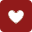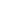×

×

×
• 剂型：
更多收起• 处方|医保：
• 厂家首字母：
• A
• B
• C
• D
• E
• F
• G
• H
• I
• J
• K
• L
• M
• N
• O
• P
• Q
• R
• S
• T
• U
• V
• W
• X
• Y
• Z
•苏宇宝贝金水[母子套装]/105ml+40g+25ml
苏州市新兴
规格： 105ml+40g+25ml
效期：2022-05-26 件装量：60
库存： 43 中包装：1加入购物车
-
+
月销:3+